# IB DP Physics: HL复习笔记9.4.2 Rayleigh Criterion Calculations

### Rayleigh Criterion Calculations

• The Rayleigh Criterion can be mathematically described by considering angular separation and single-slit diffraction
• Angular separation can be calculated using the equation:
•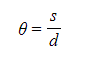•  Where:
• θ = angular separation (rad)
• s = distance between the two sources (m)
• d = distance between the sources and the observer (m)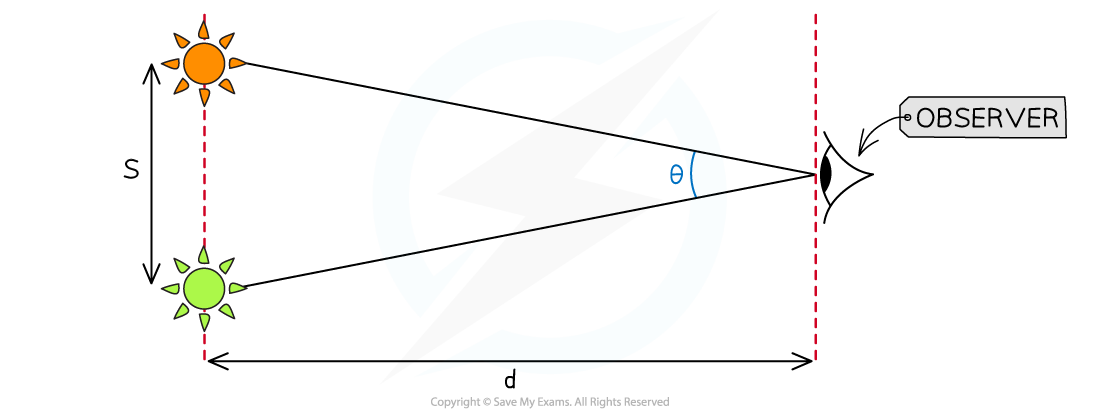Angular separation, θ, is equal to the separation, s, of two sources divided by the distance, d, between the sources and the observer

• In single slit diffraction, the first minimum occurs when the angle of diffraction is:

####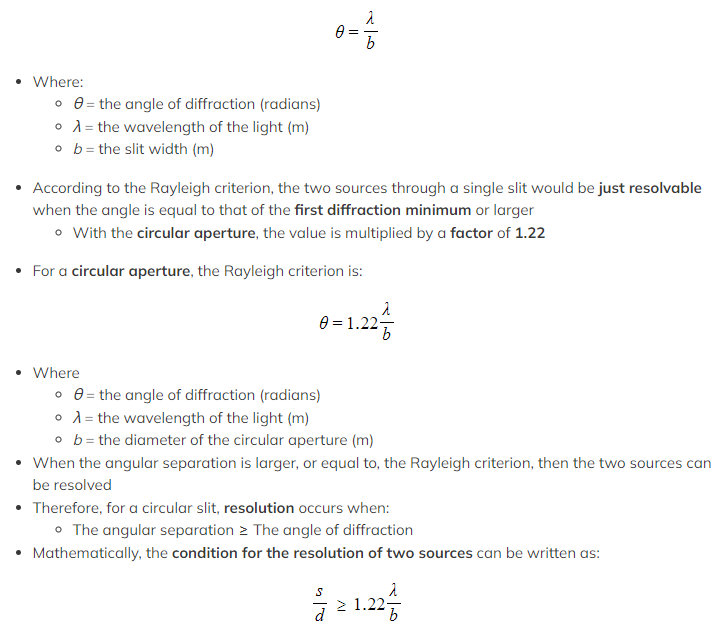Worked Example

A student looks at a helicopter in the night sky with one eye closed and can just resolve two lights as individual sources. The wavelength of both sources is 530 nm. The approximate diameter of the student’s pupil is 6.0 mm. The distance from the student to the helicopter is 6.0 km.

Determine the minimum distance between the lights.

Step 1: List the known quantities
• Wavelength of light sources, λ = 530 nm = 530 × 10−9 m
• Student pupil diameter, b = 6.0 mm = 6.0 × 10−3 m
• Distance from the helicopter light sources to the student's eye, d = 6.0 km = 6 × 103 m
Step 2: Select the relevant equation
• Since the lights can just be resolved, this is Rayleigh's criterion
• The equation needed is: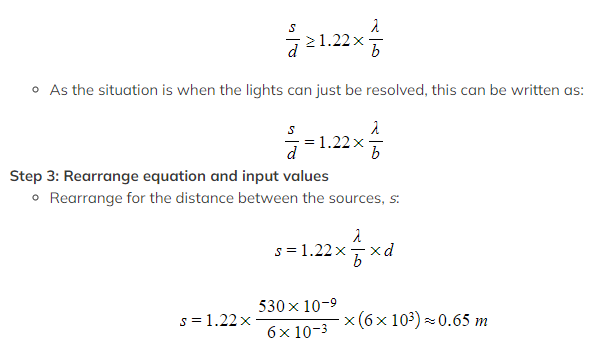Step 4: State the final answer
• The approximate distance between the light sources on the helicopter, s = 65 cm

#### Worked Example

A student views a car in the distance. It has headlights which are 1.5 m apart. The wavelength of light from the car headlights is 500 nm and the pupil diameter of the student is 4.0 mm.

Estimate the maximum distance at which the two headlights could be resolved by the student.

Step 1: List the known values
• Wavelength of light sources, λ = 500 nm = 500 × 10-9 m
• Student pupil diameter, b = 4.0 mm = 4.0 × 10-3 m
• Distance of separation between headlights, s = 1.5 m
Step 2: Select the relevant equation
• Since the answer will occur when Rayleigh’s criterion is met, the equation needed is:

####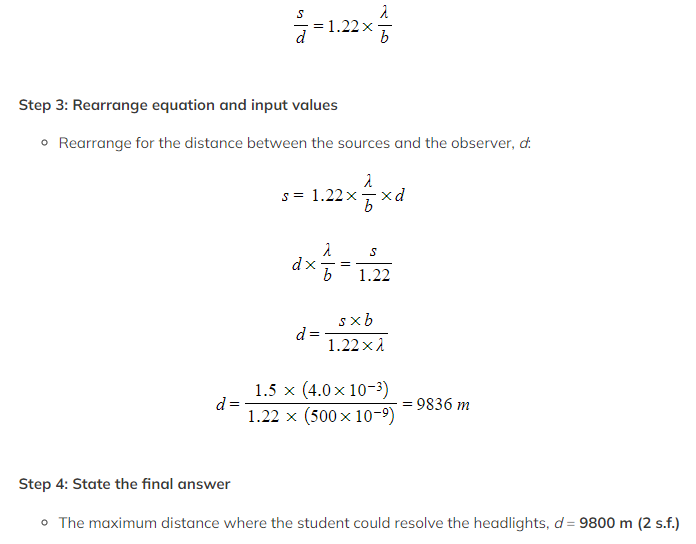Exam Tip

You might be curious where the factor of 1.22 comes from, however, the derivation of this is beyond the scope of the IB DP Physics course so just make sure you know how to use it in your calculations